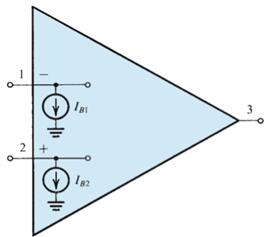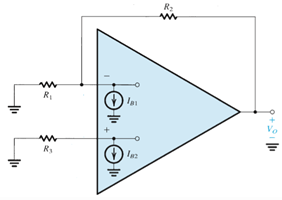Courses

# Test: DC Imperfections In Operational Amplifiers

## 10 Questions MCQ Test Electronic Devices | Test: DC Imperfections In Operational Amplifiers

Description
This mock test of Test: DC Imperfections In Operational Amplifiers for Electrical Engineering (EE) helps you for every Electrical Engineering (EE) entrance exam. This contains 10 Multiple Choice Questions for Electrical Engineering (EE) Test: DC Imperfections In Operational Amplifiers (mcq) to study with solutions a complete question bank. The solved questions answers in this Test: DC Imperfections In Operational Amplifiers quiz give you a good mix of easy questions and tough questions. Electrical Engineering (EE) students definitely take this Test: DC Imperfections In Operational Amplifiers exercise for a better result in the exam. You can find other Test: DC Imperfections In Operational Amplifiers extra questions, long questions & short questions for Electrical Engineering (EE) on EduRev as well by searching above.
QUESTION: 1

### Consider an inverting amplifier with a nominal gain of 1000 constructed from an op amp with an input offset voltage of 3 mV and with output saturation levels of ±10 V. What is (approximately) the peak sine-wave input signal that can be applied without output clipping?

Solution:

The maximum that can be sent without clipping is 10V – 1000 X 3mV or 7V.

QUESTION: 2

### (Q2 & Q.3) Consider an inverting amplifier with a nominal gain of 1000 constructed from an op amp with an input offset voltage of 3 mV and with output saturation levels of ±10 V. If the effect of VOs(input offset voltage) is nulled at room temperature (250C), how large an input can one now apply if: Q. The circuit is to operate at a constant temperature?

Solution:

Explanation: Maximum signal that will not be clipped is 10mV because 10mV X 1000 = 10V.

QUESTION: 3

### The circuit is to operate at a temperature in the range 0°C to 75°C and the temperature coefficient of VOS is 10 μV/°C?

Solution:

Since the effect is nullified at 25oC, the peak that can be sent now is given by 10 – (75-25) X 0.1 mV.

QUESTION: 4

One of the DC imperfections of the amplifiers are dc offset voltage which is

Solution:

DC offset voltage is existence of output signal even when the differential signal is zero.

QUESTION: 5

For the amplifier shown determine the value of the bias current (Ib) and input offset current (Io) respectively.Solution:

Standard mathematical expressions are used with the given variables.

QUESTION: 6

Consider the circuit shown below which reduces the impact of the input bias current. If IB1 = IB2 = Input bias current, then determine the value of R3 so that the output voltage (v0) is not impacted by the input bias current.Solution:

This will be possible when R3 has the same value as the net effect of R1 and R2.

QUESTION: 7

Consider an inverting amplifier circuit designed using an op amp and two resistors, R1 = 10 kΩ and R2 = 1 MΩ. If the op amp is specified to have an input bias current of 100 nA and an input offset current of 10 nA, find the output dc offset voltage resulting.

Solution:

Use the mathematical definition of bias current and offset current.

QUESTION: 8

(Q.8-Q.10) Consider a Miller integrator with a time constant of 1ms and an input resistance of 10 kΩ. Let the op amp have VOS (offset voltage) = 2 mV and output saturation voltages of ±12 V.​

Q. Assuming that when the power supply is turned on the capacitor voltage is zero, how long does it take for the amplifier to saturate?

Solution:

Use vO = VOS
(VOS/CR)t.

QUESTION: 9

Select the largest possible value for a feedback resistor RF so that at least ±10 V of output signal swing remains available.

Solution:

Use vO = VOS
(VOS/CR)t.

QUESTION: 10

What is the corner frequency of the resulting STC network?

Solution:

The required answer is given by 1/6 Hz.